Equilibrium

What is equilibrium?

When a chemical reaction takes place in a closed system which prevents the input or removal of any of the components involved in the reaction, the quantities of these chemicals change as some are consumed and others are formed.

Eventually this change will stop, after which the reaction composition will remain unchanged as long as the system is not disturbed. The system is then said to be in its equilibrium state, or more simply, "at equilibrium". A chemical reaction is in equilibrium when there is no tendency for the quantities of reactants and products to change.

The direction in which we write a chemical reaction (and thus which components are considered reactants and which are products) is arbitrary. Take the following two equations:

Na2CO3 + CaCl2 ->CaCO3 + 2 NaCl and CaCO3 + 2 NaCl -> Na2CO3 + CaCl2

They represent the same chemical reaction system in which the roles of the components are reversed, and both yield the same mixture of components when the change is completed. This last point is central to the concept of chemical equilibrium. It makes no difference which reactants we start with; once the reaction has run to completion, the quantities of these two components will be the same. In general, then, we can say that the composition of a chemical reaction system will tend to change in a direction that brings it closer to its equilibrium composition. Once this equilibrium composition has been attained, no further change in the quantities of the components will occur as long as the system remains undisturbed.

The equilibrium state is independent of the direction from which it is approached.

What is a reversible reaction?

Most chemical reactions are reversible. What does this mean? It means that the products of a chemical reaction, under certain conditions, can be combined to re-form the reactants. In 1045 we learned mostly about reactions that proceed to completion. These reactions are considered irreversible because the energy that would be required to reverse the process of the forward reaction is prohibitive.

Examples of "irreversible" reactions:

1. Precipitation reactions
2. Strong Acid/Base Reactions (formation of water, a covalent compound)
3. Strong Redox reactions
4. Formation of a gaseous product

All other reactions can be considered reversible.

Writing out Equilibrium Expressions:

• Even though chemical reactions that reach equilibrium occur in both directions, the reagents on the right side of the equation are assumed to be the "products" of the reaction and the reagents on the left side of the equation are assumed to be the "reactants."
• The products of the reaction are always written above the line in the numerator.
• The reactants are always written below the line in the denominator.
• For homogeneous systems, the equilibrium constant expression contains a term for every reactant and every product of the reaction.
• The numerator of the equilibrium constant expression is the product of the concentrations of the "products" of the reaction raised to a power equal to the coefficient for this component in the balanced equation for the reaction.
• The denominator of the equilibrium constant expression is the product of the concentrations of the "reactants" raised to a power equal to the coefficient for this component in the balanced equation for the reaction.

Example: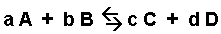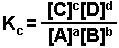The Equilibrium Constant (Kc):

The number values for equilibrium constants are tied to the nature of reactants and products in a reaction. The number values for "K" are taken from experiments measuring equilibrium concentrations. The value of K indicates the equilibrium ratio of products to reactants. In an equilibrium mixture both reactants and products co-exist.

Large K > 1 products are "favored"

K = 1 neither reactants nor products are favored

Small K < 1 reactants are "favored"

The term "favored" means that side of the equation has higher numbers of moles and higher concentrations than the other.

How to use equilibrium concentrations to determine equilibrium constants:

What is the equilibrium constant for the following reaction?

2 NO2(g) <=> 2 N2O4(g)

The concentrations at equilibrium are [NO2] = 0.025M; [N2O4] = 0.0869M. What is the equilibrium concentration for NO2 if the concentration of N2O4 is 0.12 moles/ liter? Remember the equilibrium constant is just that, a constant. It doesn't change.

Step 1: Write out the equilibrium expression: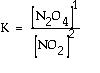Step 2: Substitute the equilibrium concentrations in the equilibrium expression. If the equilibrium constant is bigger than "1" The products are favored. This reaction tends to favor products.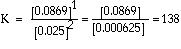Step 3: Now that the value for K is known, set up the expression for the equilibrium.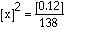Step 4: Rearrange the equation and solve for x2.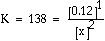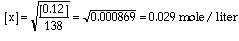The ICE Table:

An ICE table or ICE chart is a tabular system of keeping track of changing concentrations in an equilibrium reaction. ICE stands for "Initial, Change, Equilibrium". It is used in chemistry to keep track of the changes in amount of substance of the reactants and also organize a set of conditions that one wants to solve with.

Example:

For the Reaction AB <=> A + B, the ICE table would look like this:

XY
X
Y
Initial
c0
0
0
Change
-x
+x
+x
Equilibrium
c0 - x
x
x

The Equilibrium constant would then be calculated as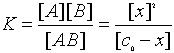This equation can then be calculated as x2 + Kx -Kc0 = 0 or if the Equilibrium constant is known then x can be calculated. In either case this is a Quadratic formula calculation.

Quadratic Formula: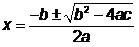Overview for Solving Problems Using the Equilibrium Constant

1. Write the chemical equation and set up the ICE table.

2. Begin filling in the ICE table by writing in the initial concentration of each species given in the problem, reactants and products. (Fill in the “I” line—the first line under the chemical equation).

3. Fill in line C of the ICE table, the change in concentrations of all species.

4. Fill in line E the ICE table, the concentration of species when the system is at equilibrium.

5. Write the equilibrium expression (this is the mathematical equation) and plug in the equilibrium concentrations as they are represented in the “E” line in the ICE table.

6. Make any assumptions if convenient.

7. Determine the numerical value of x.

8. Check any assumptions that were made in step 6. If the assumption was not valid, use the quadratic equation or the successive guess method to determine x.

9. Use the value of x to determine the equilibrium concentrations of all species. The equations written in line E of the ICE table should be used to do this.

10. Reread the problem to make sure you answered all parts of the question because by this time you may have forgotten the whole purpose of the problem!

Practice Problems:

Practice Problems

Write an equilibrium expression for each of the following reactions.

1) 3 O2 <===> 2 O3

2) N2 + 3 H2 <===> 2 NH3

3) H2 + I2 <===> 2 HI

4) PCl5 <===> PCl3 + Cl2

5) SO2 + (1/2) O2 <===> SO3